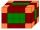# Volume - 8th grade (13y) - math problems

#### Number of problems found: 480

• Volume of ballFind the volume of a volleyball that has a radius of 4 1/2 decimeters. Use 22/7 for π
• The volumeThe volume of the sphere is 1 m3, what is its surface?
• The volume 2The volume of a cube is 27 cubic meters. Find the height of the cube.
• Water tanksA different amount of water is poured into each of the three identical vessels. In the first vessel, water fills 30% of its volume and in the second vessel 40% of its volume. The third container contains 19 liters of water. If we distributed the water fro
• Volume of sphereHow many times does the volume of a sphere increase if its radius increases 2 ×?
• Cube volumeThe cube has a surface of 384 cm2. Calculate its volume.
• Cube surface and volumeFind the surface of the cube with a volume of 27 dm3.
• Cube surfce2volumeCalculate the volume of the cube if its surface is 150 cm2.
• Area to volumeIf the surface area of a cube is 486, find its volume.
• Volume and areaWhat is the volume of a cube which has area of 361 cm2?
• Cube surface and volumeThe surface of the cube is 500 cm2, how much cm3 will be its volume?
• Surface and volumeFind the surface and volume of the rotating cone if the circumference of its base is 62.8 m and the side is 25 m long.
• 40% volume40% volume with 104 uph (units per labor hour) 8 people working. What is the volume?
• Aquarium volumeThe aquarium has a cuboid shape and dimensions a = 0.3 m, b = 0.85 m, c =? , V = ?. What volume has a body, if after dipping into the aquarium water level rises by 28 mm?
• Volume and body diagonalCalculate how much the volume and body diagonal of the cuboid decrease if we reduce each of its three edges a, b, c by 18%?
• Volume from surface areaWhat is the volume of the cube whose surface area is 96 cm2?
• Reduce of the volumeCalculate how many % reduce the volume of the cube is we reduced length of each edge by 10%.
• Volume of three cuboidsCalculate the total volume of all cuboids for which the the size of the edges are in a ratio of 1:2:3, and one of the edges has a size 6 cm.
• Scale factorA prism with a volume of 1458 mm3 is scaled down to a volume of 16mm3. What is the scale factor in fraction form?
• Volume and surfaceCalculate the volume and surface area of the cylinder when the cylinder height and base diameter is in a ratio of 3:4 and the area of the cylinder jacket is 24 dm2.

Do you have an interesting mathematical word problem that you can't solve it? Submit a math problem, and we can try to solve it.

We will send a solution to your e-mail address. Solved examples are also published here. Please enter the e-mail correctly and check whether you don't have a full mailbox.

Please do not submit problems from current active competitions such as Mathematical Olympiad, correspondence seminars etc...